Breaking News
Home / Civil Engineering / Bar Bending Schedule / HOW TO CALCULATE STEEL QUANTITY FOR SLAB WITH BAR BENDING SCHEDULE

# HOW TO CALCULATE STEEL QUANTITY FOR SLAB WITH BAR BENDING SCHEDULE

## HOW TO CALCULATE STEEL QUANTITY FOR SLAB WITH BAR BENDING SCHEDULE

### Hi before going to calculate bar bending schedule for slab you should know to find your slab is whether one-way slab or two-way slab. If not its ok we will guide through this

 One Way Slab Ly/Lx > 2 Two Way Slab Ly/Lx < 2

### in Two-way slab Main bars (cranked bars) are provided in both directions.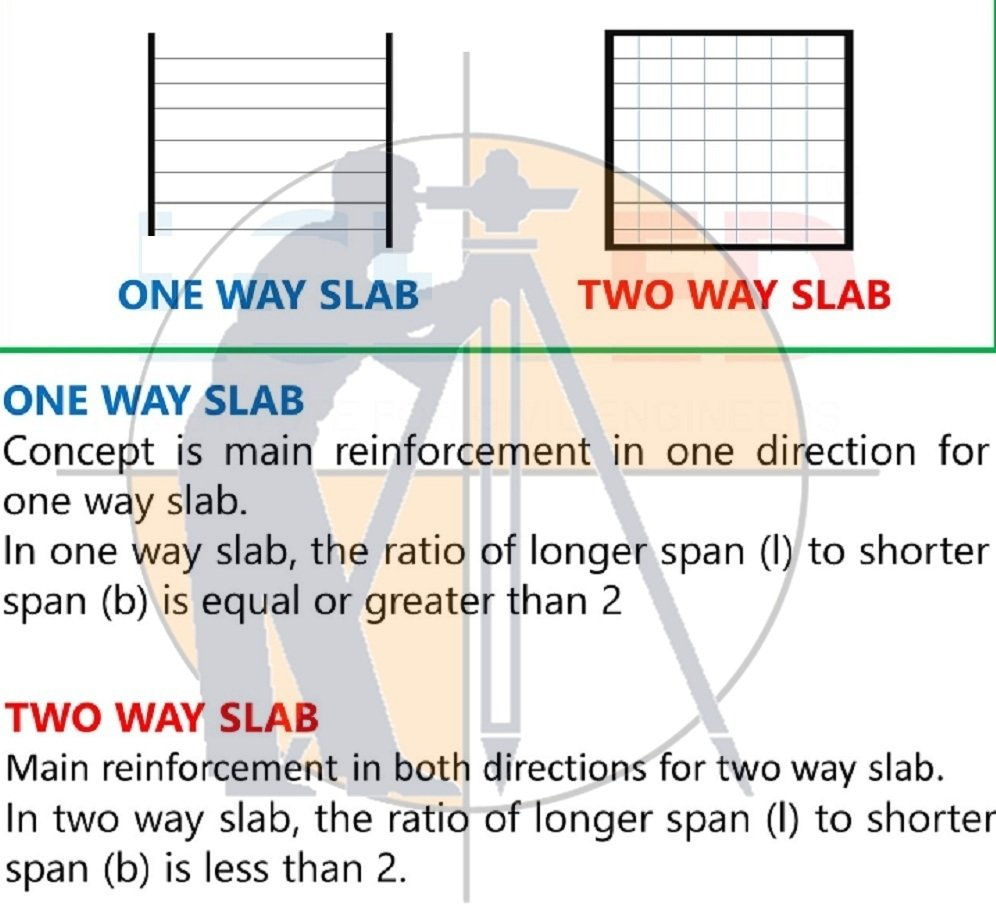### therefore,

D = Depth of Slab – Top cover – Bottom cover

### Extra Bars: The extra bar is provided at the bottom of Cranked bars to maintain the framework of the slab. The length of Extra bar is L/4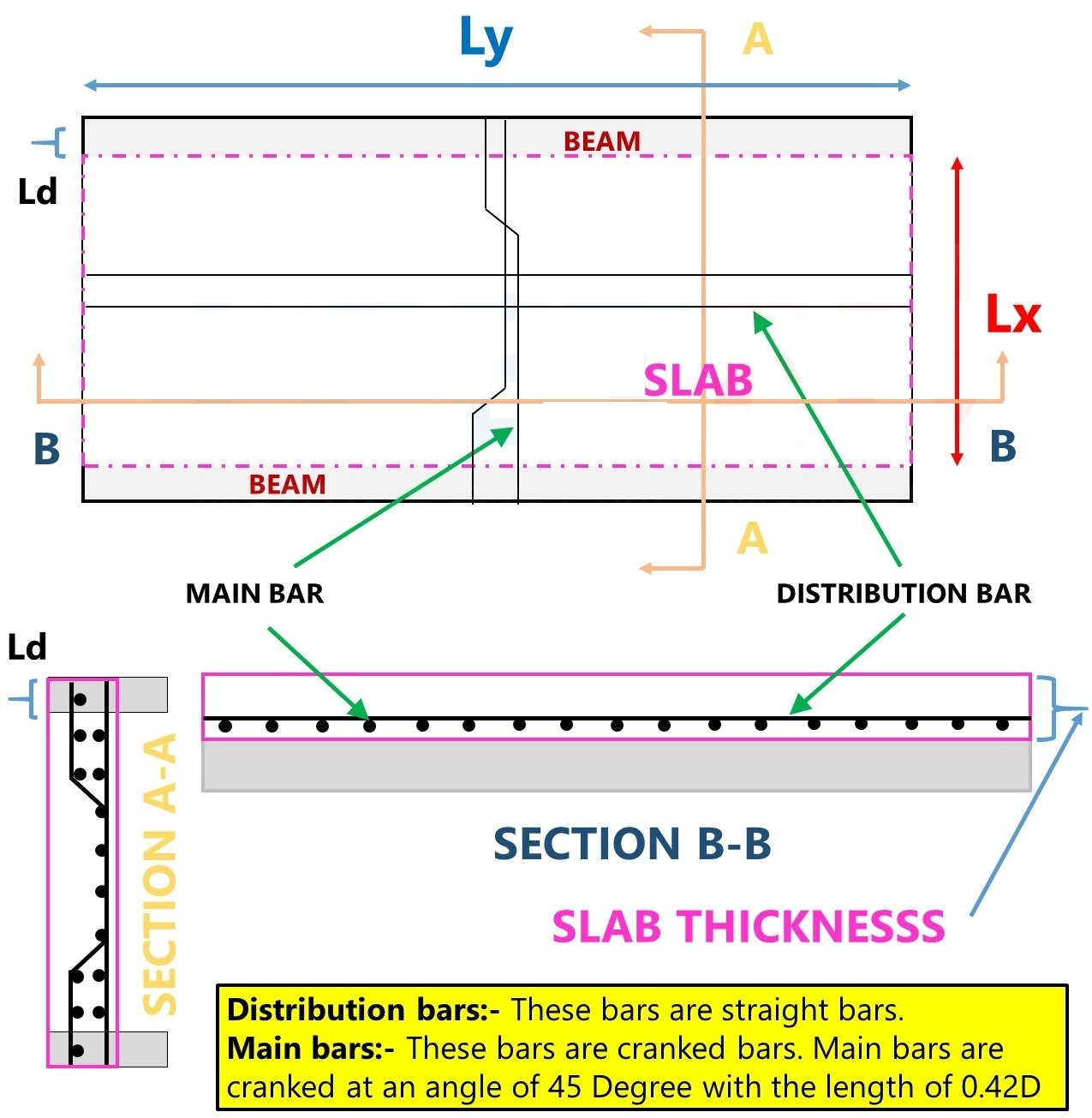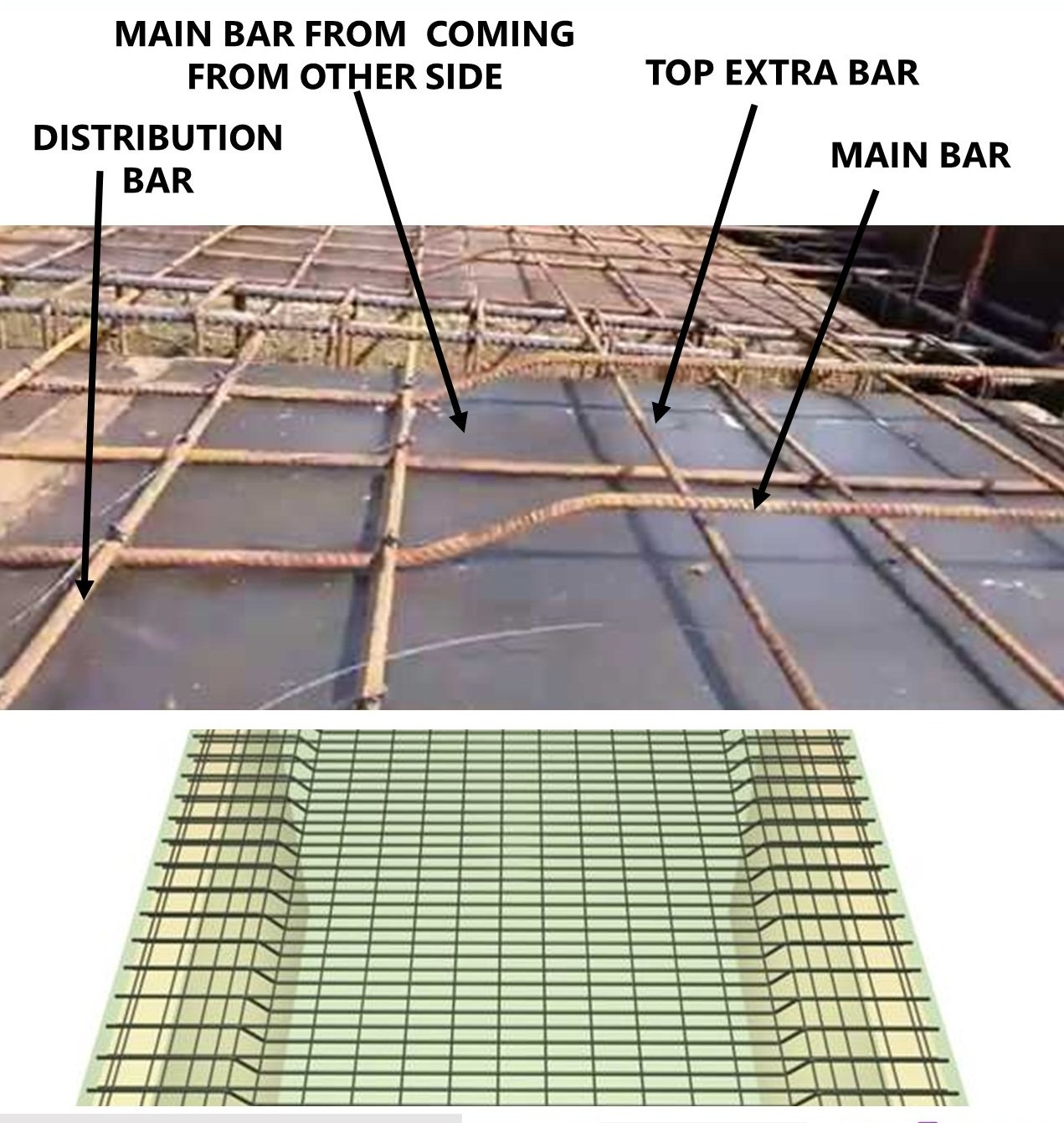### OK We Think You Got A Clear Picture Of Slab Reinforcements Now Take Look Of This Detailing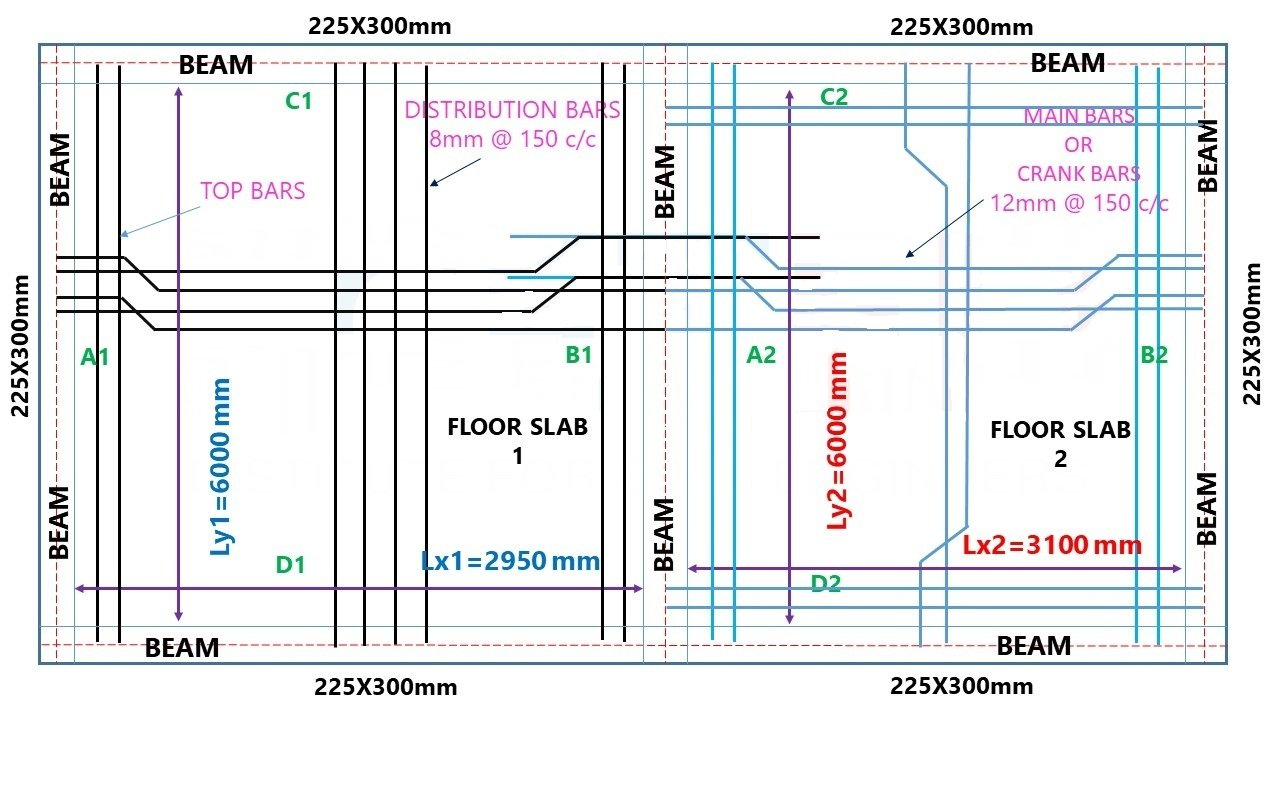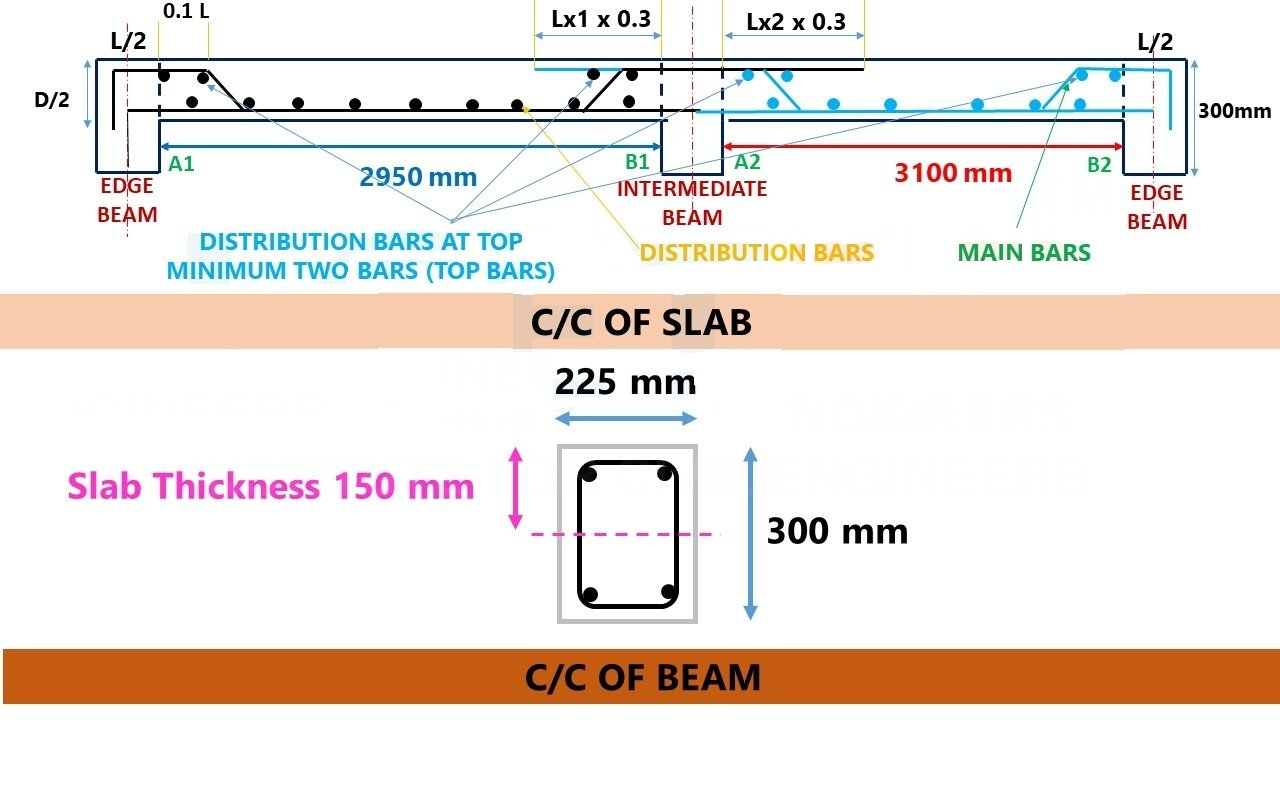### SIZE OF FLOOR SLAB 1

Length= 2950mm

Beam size = 225x300mm

Main bars are 12 mm in diameter @ 150 mm centre to centre spacing

Distribution bars are 8 mm in diameter @ 150 mm centre to centre spacing. (Main Bar & Distribution Bar Difference)

Top and Bottom Clear Cover is 25 mm

Thickness of Slab – 150 mm

### How to Calculate Staircase Concrete & Bar Bending Schedule And Staircase Reinforcement Details

Therefore, To Find Its One Way Or Two Way Slab

Lx = 2950mm (shorter span)

Ly = 6000mm (Larger span)

Ly/Lx= 6000/2950 = 2.033

 One Way Slab Ly/Lx > 2

Hence its one-way slab

So, We need to find

• Main bar for shorter span
• Distribution bar for longer span
• Top bar for main rod on both sides for critical structure

### LCETED TRIVIA

1. Main reinforcement bar is normally used at the bottom of the slab.

Distribution bars are placed on the top of the main bar.

1. Main bar is used in shorter direction but distribution bar is used in longer span.
2. Higher dimension bar is used as main reinforcement bar.

Lower dimension bar is used as distribution bars.

1. Main reinforcement bar is used to transfer the bending moment to beams.

Distribution bars are used to resist the shear stress, and cracks developed at the top of the slab

### STEP 1

Find Cutting Length Of Main Bars For Floor Slab 1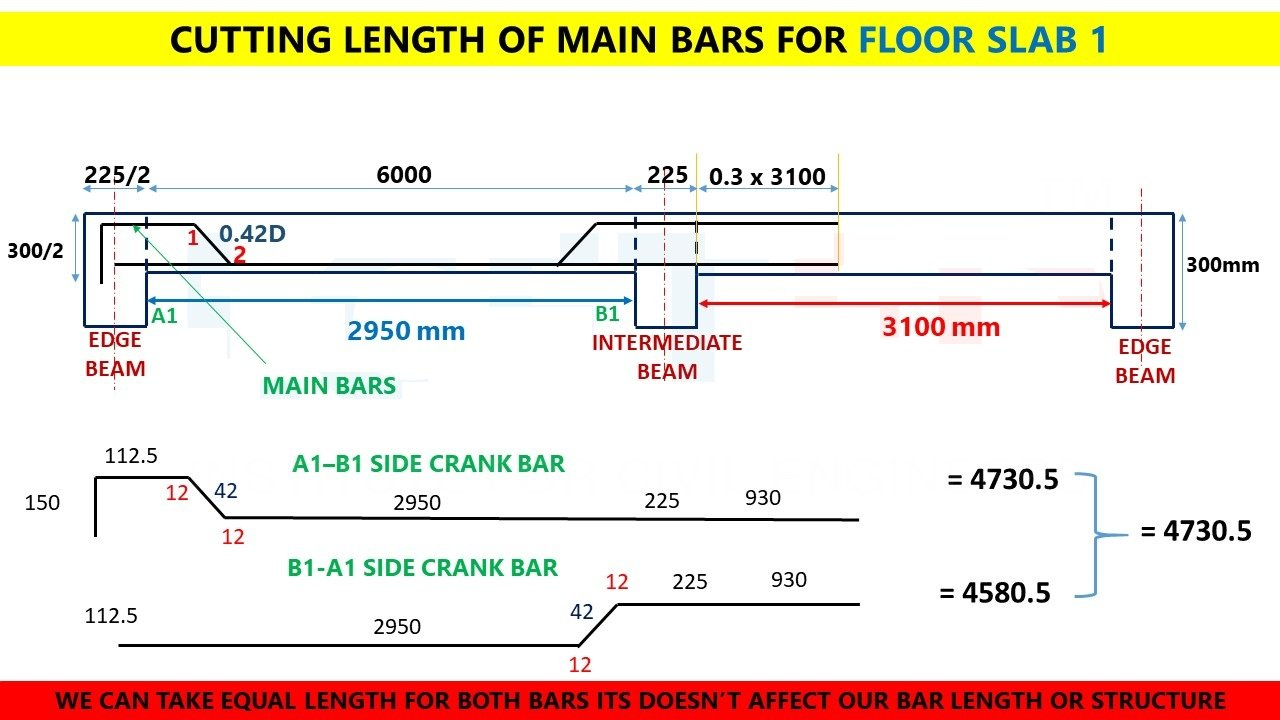### D = Slab thickness – 2 side clear cover (top cover + bottom cover)

D = 150 – (2 x 25) = 100mm

Inclined length = 0.42 x 100 = 42mm

45° bend = 1d

d = diameter of bar

45° bend = 1 x 12 = 12mm

Intermediate slab

L = 3100mm

### STEP 2

No Of Bars Required For Cutting Main Bars

### No of bars = (opp length/spacing) + 1

Opposite length of bar = 6000

Spacing = 150mm c/c as per given details

### STEP 3

Total Length Of Bar Required For Main Bars Of Floor Slab 1

= no of bar x total length of bar

= 41 x 4.80 = 196.8m = 197m

### STEP 4

Find Cutting Length Of Distribution Bars For Floor Slab 1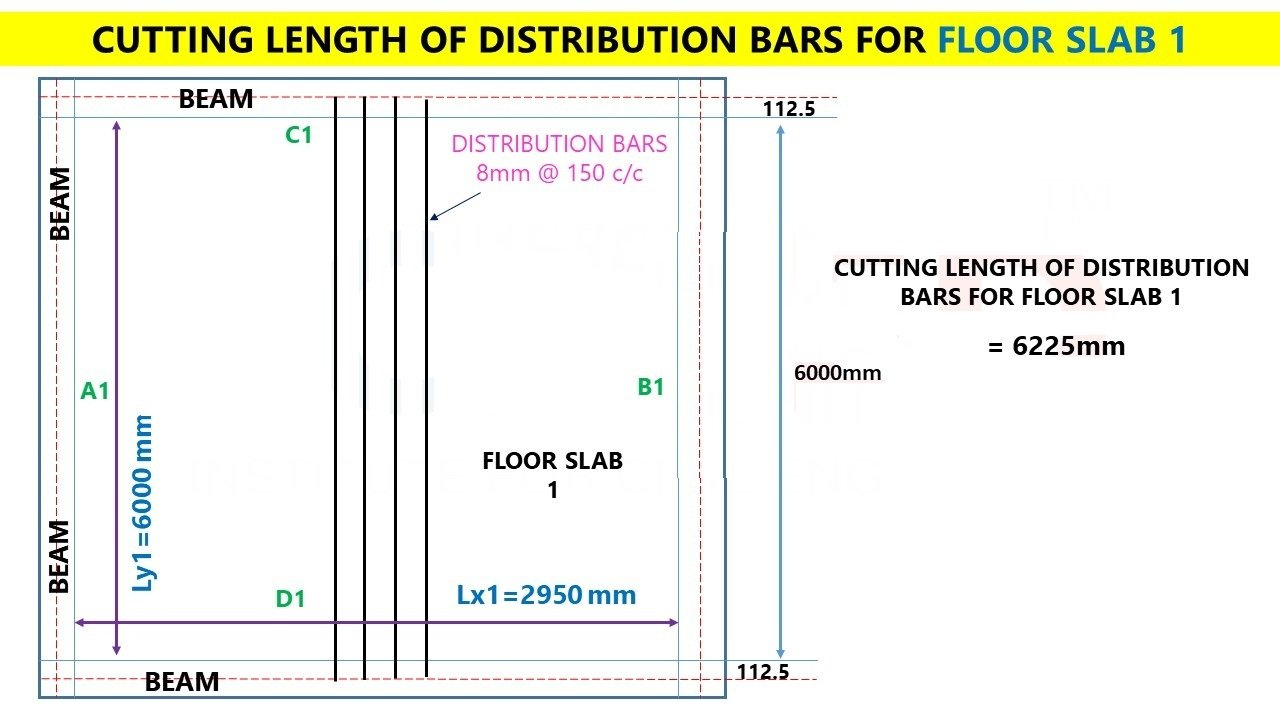Length of distribution bars = (breadth of beam/2) + length of longer span + (breadth of beam/2)

Length of distribution bars = (225/2) + 6000 +(225/2) = 6225mm

Length of distribution bars = 6.225m

### Estimate this Slab (Drawing Included)

STEP 5

No Of Bars Required For Cutting Distribution Bars

### No of bars = (opp length/spacing) + 1

Opposite length of bar = 2950

Spacing = 150mm c/c as per given details

### STEP 6

Total Length Of Bar Required For Distribution Bars Of Floor Slab 1

= No Of Bar X Total Length Of Bar

= 21 x 6.225 = 130.725m

### STEP 7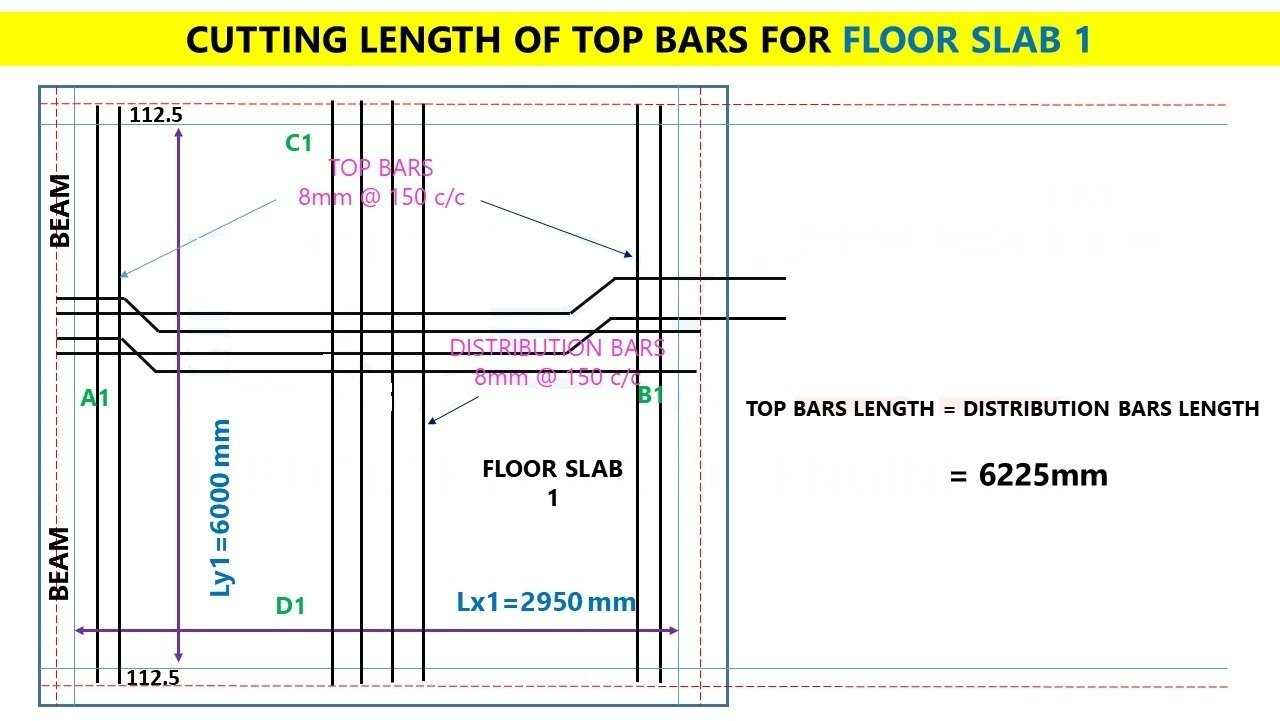### Top Bar (Extra): Top Bars Are Provided At The Top Of Main Bar As Critical Length (L/4) Area

Number of top bars = (Lx/4) / spacing + 1

Number of top bars = ((2950/4) / 150) + 1 =5.91 = 6 nos of bars x 2 sides = 12 nos

Number of top bars = 12 nos of bars

### STEP  8

Total Length Of Bar Required For Top Bars Of Floor Slab 1

### Length of top bars = Length of Distribution Bars

Cutting Length Of top Bars = 6.225m

Total length of top bars = 6.225 x 10 = 27.25m

Length= 3100mm

### Therefore,

Lx shorter span = 3100mm

Ly larger span = 6000mm

Beam size = 225x300mm

Main bars are 12 mm in diameter @ 150 mm centre to centre spacing

Distribution bars are 8 mm in diameter @ 150 mm centre to centre spacing. (Main Bar & Distribution Bar Difference)

Top and Bottom Clear Cover is 25 mm

Consider Development length as 40 d

Thickness of Slab – 150 mm

### Therefore, To Find Its One Way Or Two Way Slab

Lx = 3100mm (shorter span)

Ly = 6000mm (Larger span)

Ly/Lx= 6000/3100 = 1.93

### Hence its Two-way slab

So, We need to find

• Main bar for shorter span
• Main bar for longer span
• Top bar for main rod on both sides for critical structure

### STEP 1

Find Cutting Length Of Main Bars For Floor Slab 2 (A2-B2 direction)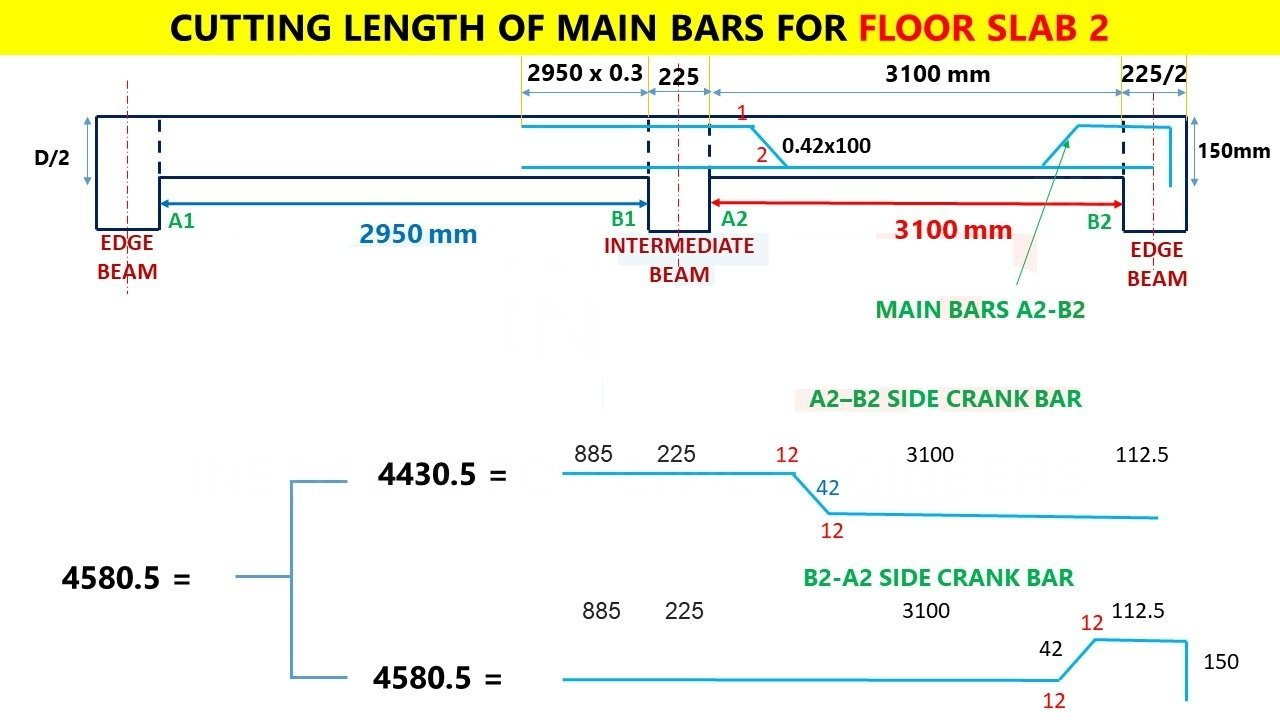### D = Slab thickness – 2 side clear cover (top cover + bottom cover)

D = 150 – (2 x 25) = 100mm

Inclined length = 0.42 x 100 = 42mm

### 45° bend = 1d

d = diameter of bar

45° bend = 1 x 12 = 12mm

L = 3000mm

### Therefore,

Length Of Main Bars (A2-B2) = (300/2) + (225/2) + (1 x 42) – (12 x 2)  + 3100 + 300 + (0.3 x 3000) = 4580.5

### STEP 2

No Of Bars Required For Cutting Main Bars

### No of bars = (opp length/spacing) + 1

Opposite length of bar = 6000

Spacing = 150mm c/c as per given details

### STEP 3

Total Length Of Bar Required For Main Bars Of Floor Slab 2

= no of bar x total length of bar

= 41 x 4.6= 188.6m = 190m

### STEP 4

Find Cutting Length Of Main Bars For Floor Slab 2 (C2-D2 direction)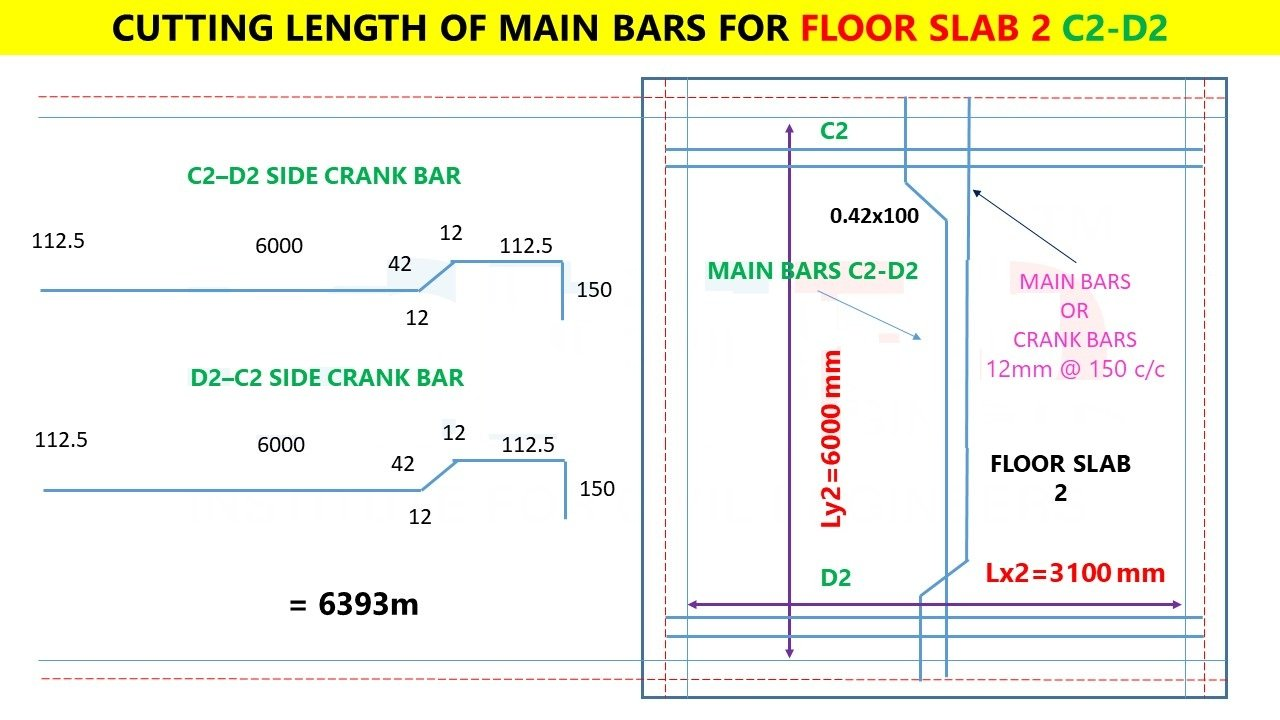### D = Slab thickness – 2 side clear cover (top cover + bottom cover)

D = 150 – (2 x 25) = 100mm

Inclined length = 0.42 x 100 = 42mm

### 45° bend = 1d

d = diameter of bar

45° bend = 1 x 12 = 12mm

L = 3000mm

### Therefore,

Length Of Main Bars (C2-D2) = (300/2) + (225/2) + (1 x 42) – (12 x 2)  + 6000 + (225/2) = 6393m

### STEP 5 e

No Of Bars Required For Cutting Main Bars

### No of bars = (opp length/spacing) + 1

Opposite length of bar = 3100

Spacing = 150mm c/c as per given details

### STEP 6

Total Length Of Bar Required For Main Bars Of Floor Slab 2

= no of bar x total length of bar

= 22 x 6.4= 140.8m = 141m

### Top Bar (Extra) (A2-B2): Top Bars Are Provided At The Top Of Main Bar As Critical Length (L/4) Area

Number of top bars = (Ly/4) / spacing + 1

Number of top bars = ((6000/4) / 150) + 1 = 11 nos of bars x 2 sides = 22 nos

### Number of top bars = 22 nos of bars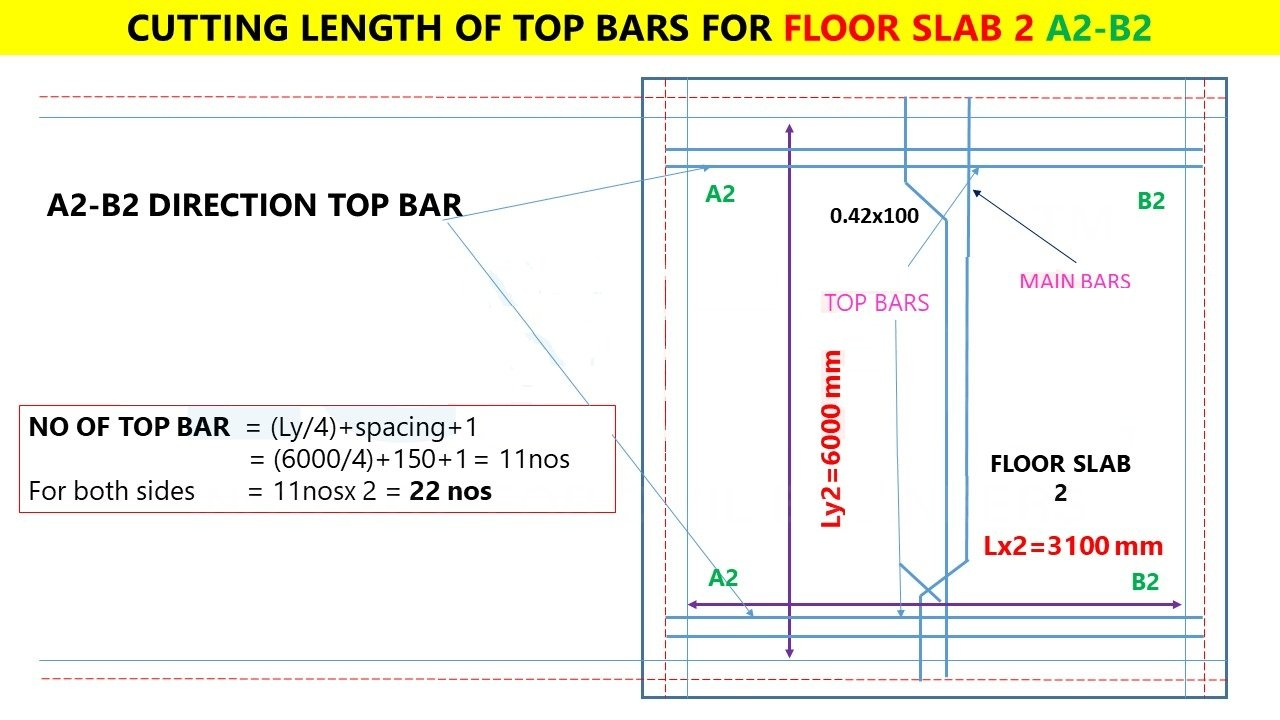### Top Bar (Extra) (C2-D2): Top Bars Are Provided At The Top Of Main Bar As Critical Length (L/4) Area

Number of top bars = (Lx/4) / spacing + 1

Number of top bars = ((3100/4) / 150) + 1 =6.16 = 7 nos of bars x 2 sides = 14 nos

### Number of top bars = 14 nos of bars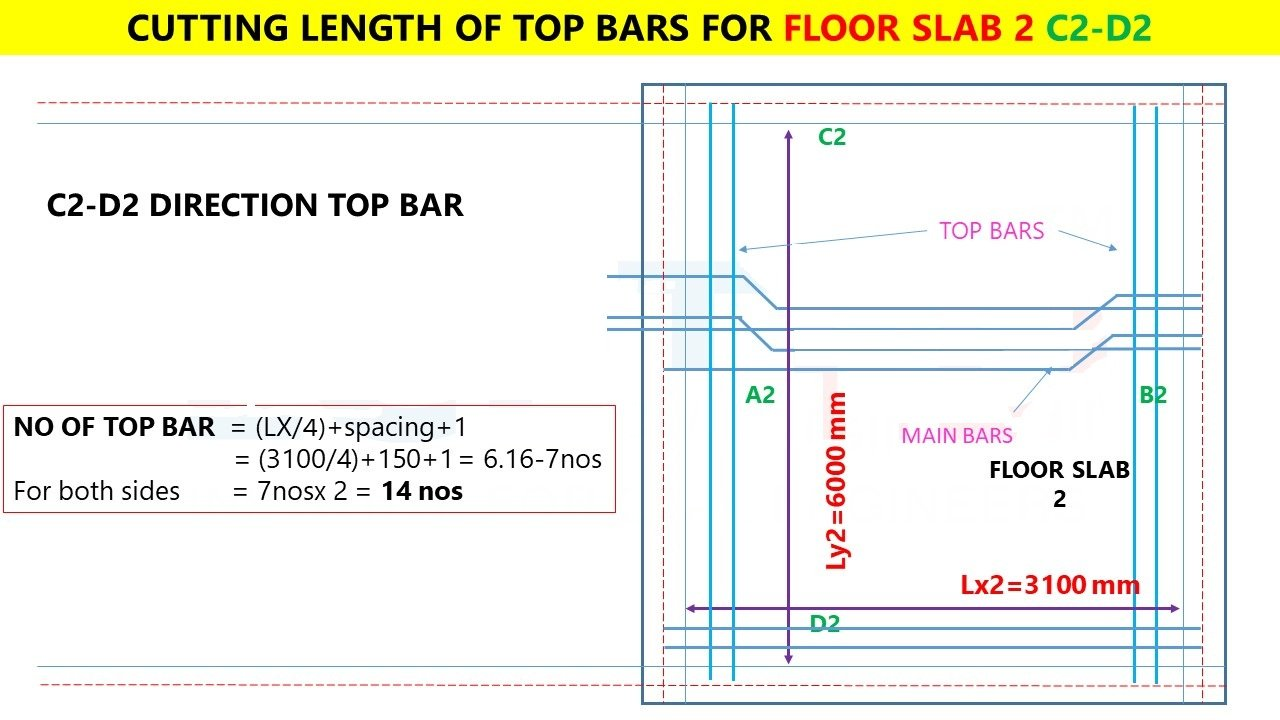### STEP  8

Total Length Of Bar Required For Top Bars Of Floor Slab 2 (A2-B2)

### Length of top bars = Length of Opposite Bars (C2-D2)

Cutting Length Of top Bars = 3.325m

Total length of top bars = 3.325 x 14 = 46.55m

### Total length of top bars of Floor Slab 2= 47 m of 8mm rod

Total Length Of Bar Required For Top Bars Of Floor Slab 2 (C2-D2)

### Length of top bars = Length of Opposite Bars (A2-B2)

Cutting Length Of top Bars = 6.225m

Total length of top bars = 6.225 x 14 = 87.15m

### Checklist For R.C.C Slab & Beams

RESULT,

FLOOR SLAB 1,

Main Bars = 185m Of 12mm Rod

Distribution Bars = 131m Of 8mm Rod

Top Bars = 63m Of 8mm Rod

### FLOOR SLAB 2,

Main Bars = 190m of 12mm rod

Main Bars = 141m Of 12mm Rod

Top Bars = 88 m of 8mm rod (A2-B2)

Top Bars = 47m of 8mm rod (C2-D2)

### TOTAL

12mm rod required = 516m

8mm rod required = 329m

### THANKS.